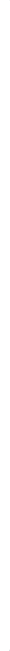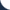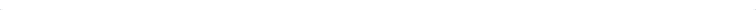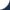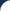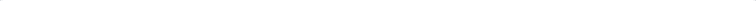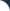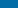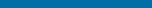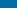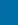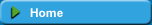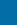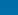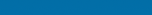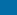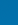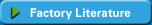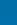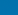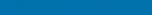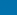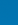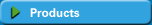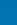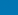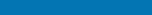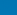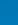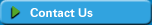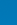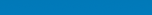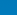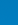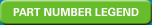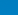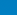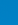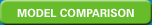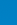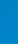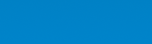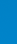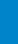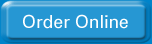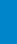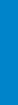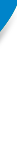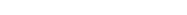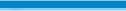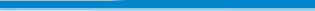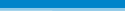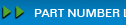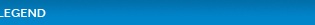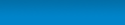Pedrollo USA Part Number Legend HP VOLTAGE 1PH / 3 PH HERTZ FEATURES 025= 1/4 A=115 1 5=50 S =STANDARD 03= 1/3 B=200 3 6=60 P = Pre- Assembled 05= 1/2 C=230 A= 30' Cord 07= 3/4 D=460 B= 60' Cord 10= 1 E=575 C=90' Cord 15= 1-1/2 F=115/230 D= 120' Cord 20= 2 H=230/460 E=120' Cord 30= 3 F= 15' Cord + GM 50= 5 G= 30' Cord + GM 75= 7-1/2 H= 45' Cord + GM 100= 10 I= 60' Cord + GM 125= 12-1/2 J= 90' Cord + GM 150= 15 K= 15' Cord + PBFS 200= 20 L= 30'  Cord + PBFS 250= 25 M= 45' Cord+ PBFS N= 60' Cord + PBFS O= 75' Cord + PBFS W=Silicon Carbide Seal

GM= Magnetic Float Switch
PBFS= Piggy Back Float Switch

 Example CP150H36S CP= Series 150= 15 HP H=230/460 3= Phase 6= 60 HZ S= StandardPedrollopumpdistributor.com. is an independent supplier of products. Trademarks, brand names and logos are the property of each manufacturer or its affiliates and are solely used to identify their respective products.